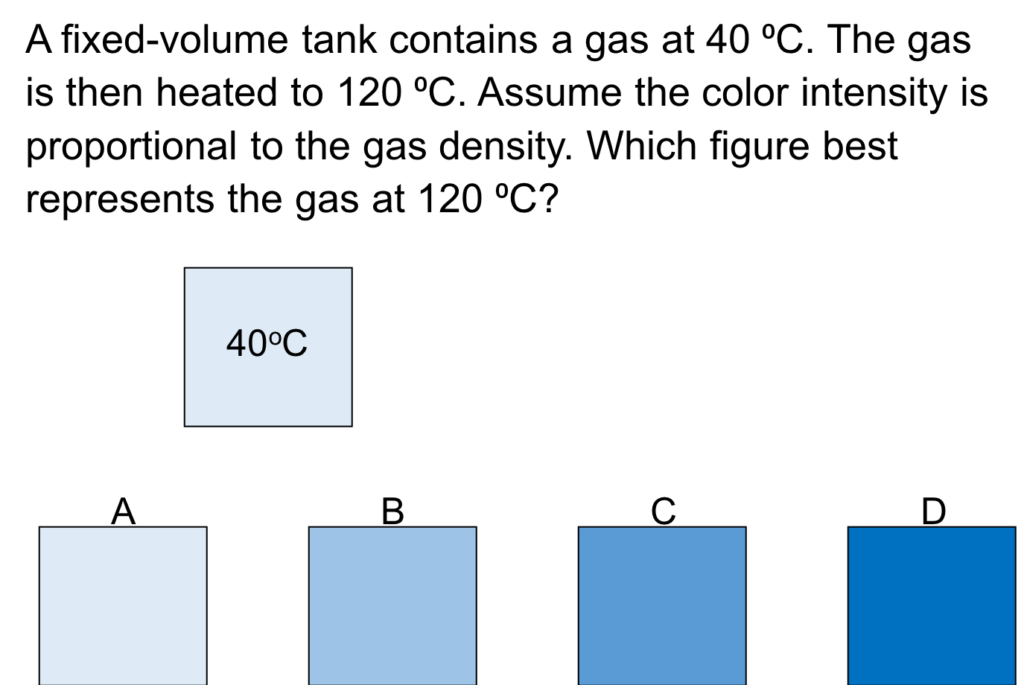LearnChemE

#### Ideal Gas Law: ConcepTest and Example Problem

Try to answer this ConcepTest and solve the example problem before using this module. Studies show that trying to answer the questions before studying material improves learning and retention. We suggest that you write down the reasons for your answers. By the end of this module, you should be able to answer these on your own. Answers will be given at the end of this module.##### Example Problem 1

Calculate the volume of water vapor using the ideal gas law. Determine how much error is made by assuming water is an ideal gas by comparing calculated volumes to volumes from the steam tables.

1. At 500°C and 0.5-MPa pressure, water’s specific volume is 0.7109 m3/kg.
2. At 500°C and 17.0-MPa pressure, water’s specific volume is 0.0482 m3/kg.